Odometer

The odometer is driven by rotation of the wheel whose diameter is 65 cm. After how many rotations the wheel turns counter to next kilometer?

Result

n =  489.7

Solution:Leave us a comment of example and its solution (i.e. if it is still somewhat unclear...):Be the first to comment!Next similar examples:

1. Bicycle wheelAfter driving 157 m bicycle wheel rotates 100 times. What is the radius of the wheel in cm?
2. Bicycle wheel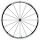Bicycle wheel diameter is 62 cm. How many times turns the bicycle on the road 1 km long?
3. Velocipede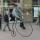The front wheel of velocipede from year 1880 had a diameter 1.8 m. If the front wheel turned again one then rear wheel 6 times. What was the diameter of the rear wheel?
4. CircleWhat is the radius of the circle whose perimeter is 6 cm?
5. Circle - simpleThe circumference of a circle is 930 mm. How long in mm is its diameter?
6. Date game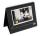Let now is Wednesday. What day is after 248 days? Write the result as a number: 1=Monday, 2=Tuesday, 3=Wednesday, 4=Thursday, 5=Friday, 6=Saturday, 7=Sunday?
7. Crates 2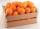One crate will hold 50 oranges. If Bob needs to ship 932 oranges, how many crates will he need?
8. The resultHow many times I decrease the number 1632 to get the result 24?
9. Math classificationIn 3A class are 27 students. One-third got a B in math and the rest got A. How many students received a B in math?
10. Fractions 4How many 2/3s are in 6?
11. Sweets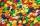Mom bought box of sweets for their children.Whole package of 100 sweets divided among 4 their children so that each child receives the most and for she remains the least sweets. How many sweets left for mom.
12. Clock handsThe second hand has a length of 1.5 cm. How long does the endpoint of this hand travel in one day?
13. Circles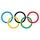For the circle c1(S1; r1=146 cm) and c2(S2; r2 = 144 cm) is distance of centers |S1S2| = 295 cm. Determine the distance between the circles.
14. Pizza 5You have 2/4 of a pizza and you want to share it equally between 2 people how much pizza does each person get?
15. Product of two fractionsProduct of two fractions is 9 3/5 . If one of the fraction is 9 3/7. Find the other fraction.
16. Circle - easy 2The circle has a radius 6 cm. Calculate:
17. Circle from string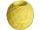Martin has a long 628 mm string . He makes circle from it. Calculate the radius of the circle.